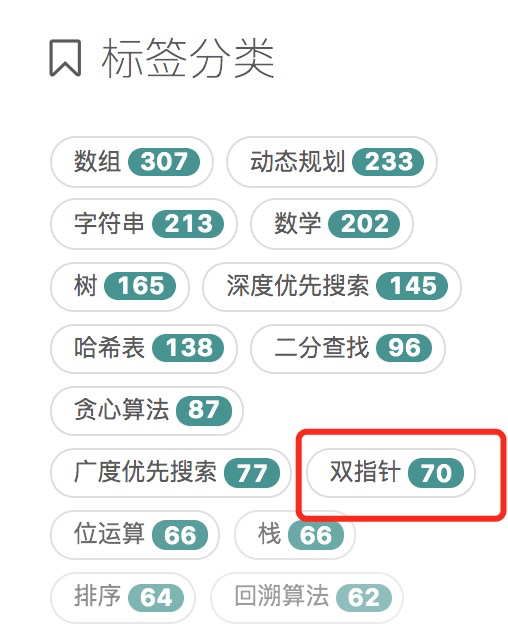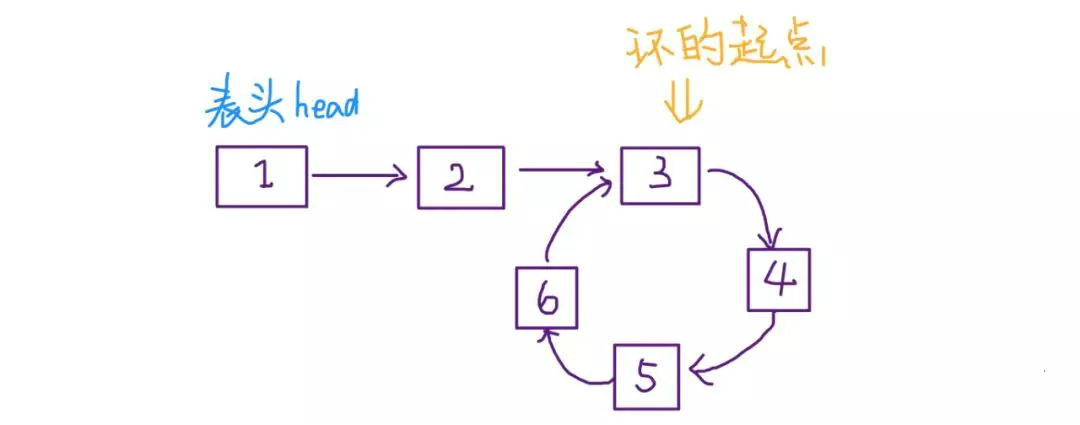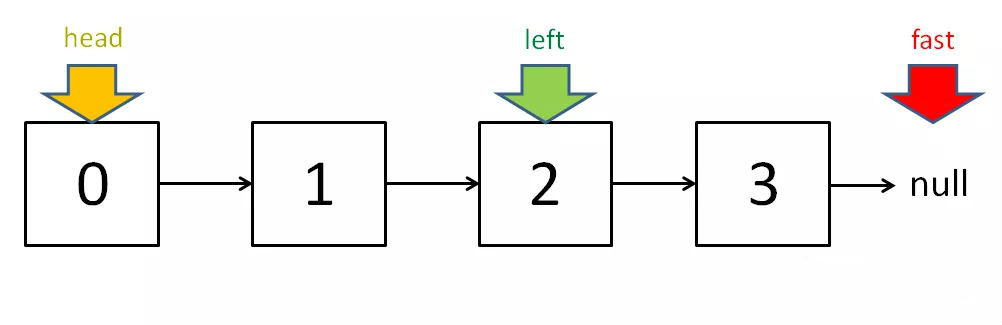# 什么是双指针# 对撞指针

public void find (int[] list) {
var left = 0;
var right = list.length - 1;

//遍历数组
while (left <= right) {
left++;
// 一些条件判断 和处理
... ...
right--;
}
}

## 算法实例

### 344. 反转字符串

输入：["h","e","l","l","o"]

输入：["H","a","n","n","a","h"]

#### 解答

class Solution {
public void reverseString(char[] s) {
if (s.length == 0 || s.length == 1) return ;
int left = 0;
int right = s.length-1;
while (left <right) {
char temp = s[left];
s[left++] = s[right];
s[right--] = temp;
}
return ;
}
}

### 209. 长度最小的子数组

输入：s = 7, nums = [2,3,1,2,4,3]

#### 解答

class Solution {
public int minSubArrayLen(int s, int[] nums) {
int right =0;
int left=0;
int sum =0;
int len =Integer.MAX_VALUE;
while(right < nums.length) {
sum+=nums[right];
while (sum >=s) {
len = Math.min(right -left+1,len);
sum -= nums[left];
left++;
}
right++;
}
if (len == Integer.MAX_VALUE) return 0;
return len;

}
}

# 快慢指针

LeetCode 141.环形链表为例,，判断给定链表中是否存在环，可以定义快慢两个指针，快指针每次增长一个，而慢指针每次增长两个，最后两个指针指向节点的值相等，则说明有环。就好像一个环形跑道上有一快一慢两个运动员赛跑，如果时间足够长，跑地快的运动员一定会赶上慢的运动员。

## 算法示例

### 1、判定链表中是否含有环

boolean hasCycle(ListNode head) {
return false;
}

boolean hasCycle(ListNode head) {
ListNode fast, slow;
while(fast != null && fast.next != null) {
fast = fast.next.next;
slow = slow.next;
if (fast == slow)
return true;
}
return false;
}

### 2、已知链表中含有环，返回这个环的起始位置ListNode detectCycle(ListNode head) {
ListNode fast, slow;
while (fast != null && fast.next != null) {
fast = fast.next.next;
slow = slow.next;
if (fast == slow)
break;
}

while (slow != fast) {
fast = fast.next;
slow = slow.next;
}
return slow;
}

### 3、寻找链表的中点

ListNode slow, fast;
while (fast != null && fast.next != null) {
fast = fast.next.next;
slow = slow.next;
}
// slow 就在中间位置
return slow;### 4、寻找链表的倒数第 k 个元素

ListNode slow, fast;
while (k-- > 0)
fast = fast.next;

while (fast != null) {
slow = slow.next;
fast = fast.next;
}
return slow;

# 滑动窗口算法

### 参考文章：

https://www.cnblogs.com/kyoner/p/11087755.html

https://zhuanlan.zhihu.com/p/71643340

posted @ 2020-08-23 16:59  huansky  阅读(11775)  评论(0编辑  收藏  举报